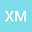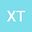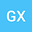Almost sharp global well-posedness for the defocusing Hartree equation with radial data in $\mathbb R^5$
•••• +1
• Xingjian Mu,
• Xingdong Tang,
• Guixiang Xu,
• Jianwei Yang
Xingjian Mu
Beijing Normal University

Corresponding Author:[email protected]

Author ProfileXingdong Tang
Nanjing University of Information Science and Technology
Author ProfileGuixiang Xu
Beijing Normal University
Author ProfileJianwei Yang
Beijing Institute of Technology
Author Profile## Abstract

We show global well-posedness and scattering for the defocusing, energy-subcritical Hartree equation \begin{equation*} iu_t + \Delta u =F(u), (t,x)\in\mathbb{R}\times\mathbb{R}^5 \end{equation*} where $F(u)= \big( V* |u|^2 \big) u$, $V(x)=|x|^{-\gamma}$, $3< \gamma< 4$, and initial data $u_0(x)$ is radial in almost sharp Sobolev space $H^{s}\left(\R^5\right)$ for $s>s_c=\gamma/2-1$. Main difficulty is the lack of the conservation law. The main stategy is to use I-method together with the radial Sobolev inequality, the interaction Morawetz estimate, long-time Strichartz estimate and local smoothing effect to control the energy transfer of the solution and obtain the increment estimate of the modified energy $E(Iu)(t)$.